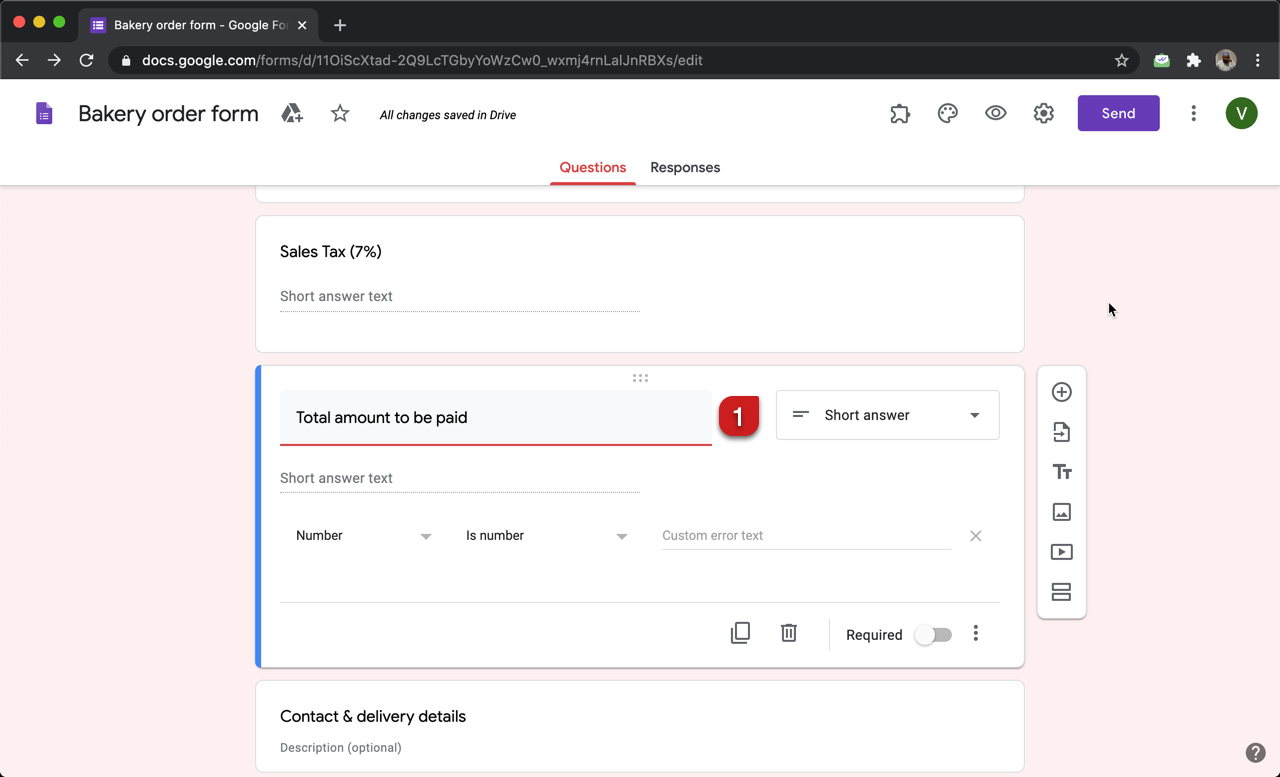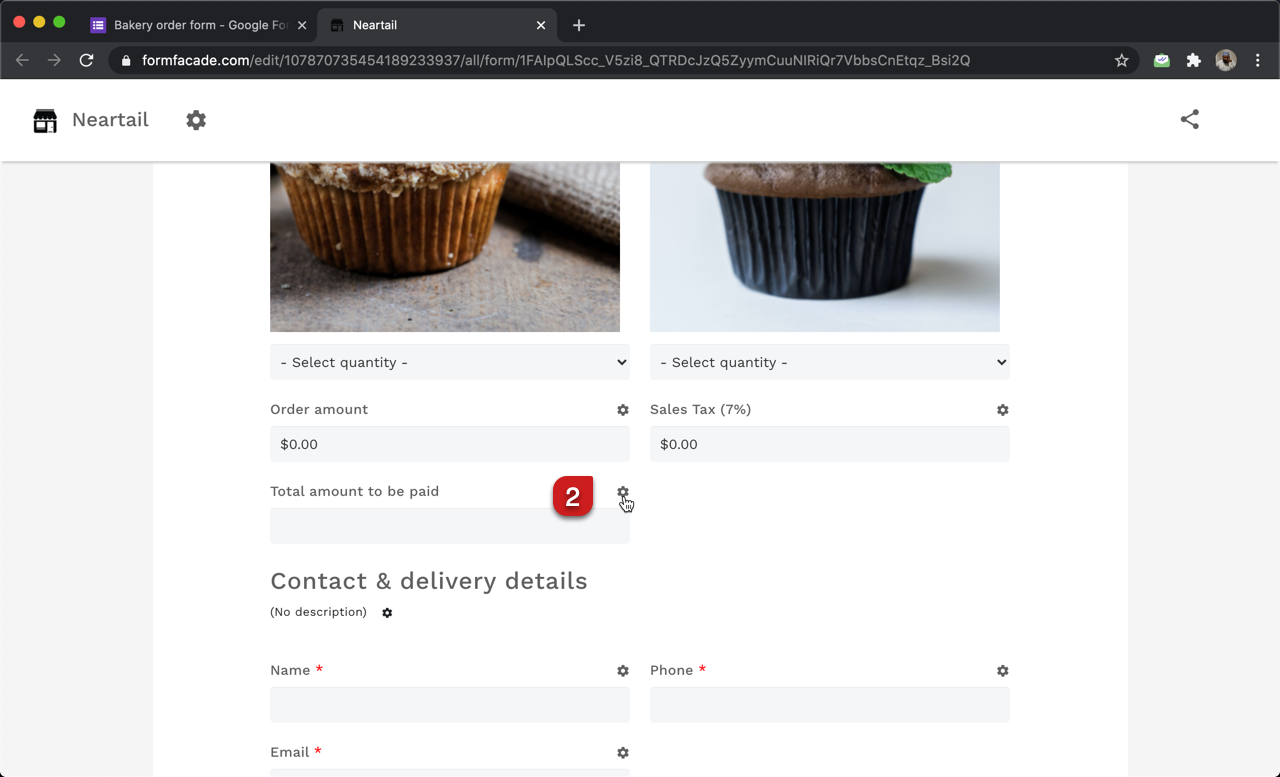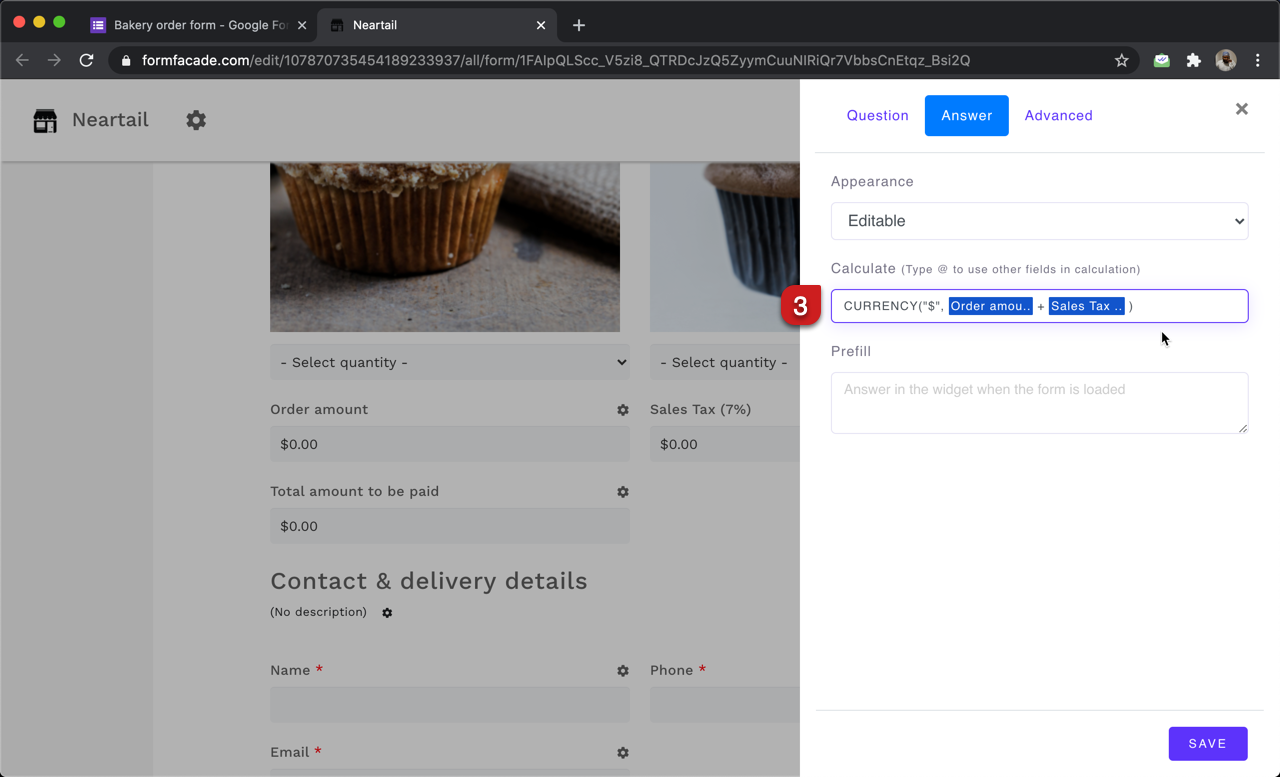### You can add a short answer question in Google Forms and then write the formula in Formfacade to automatically calculate the taxes based on order amount.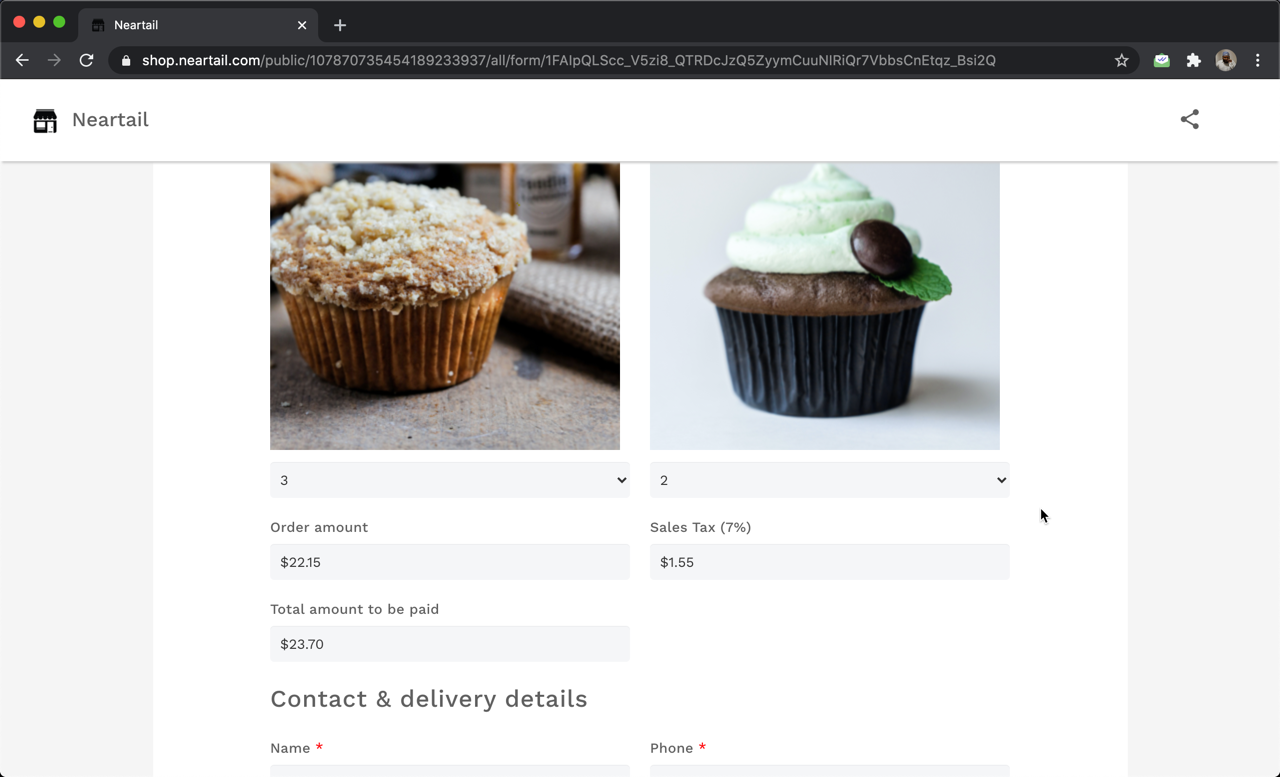#### How to calculate the taxes based on order amount?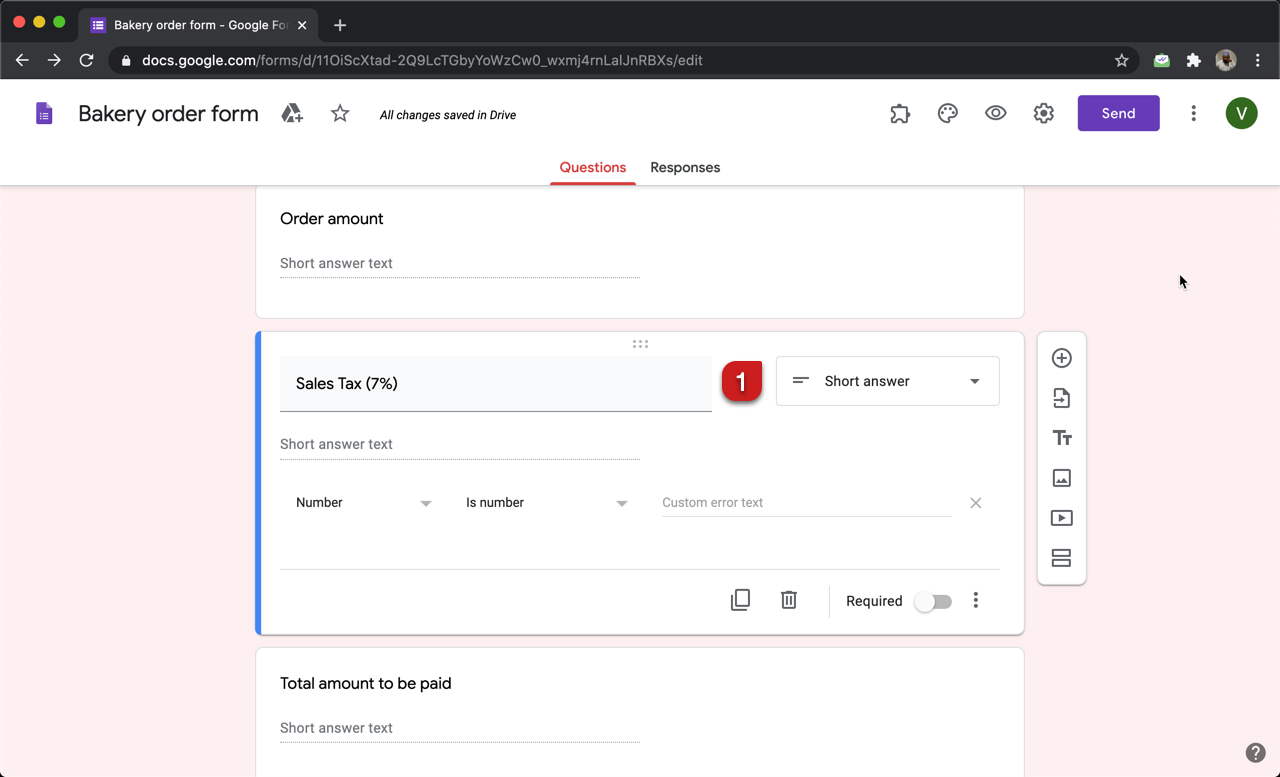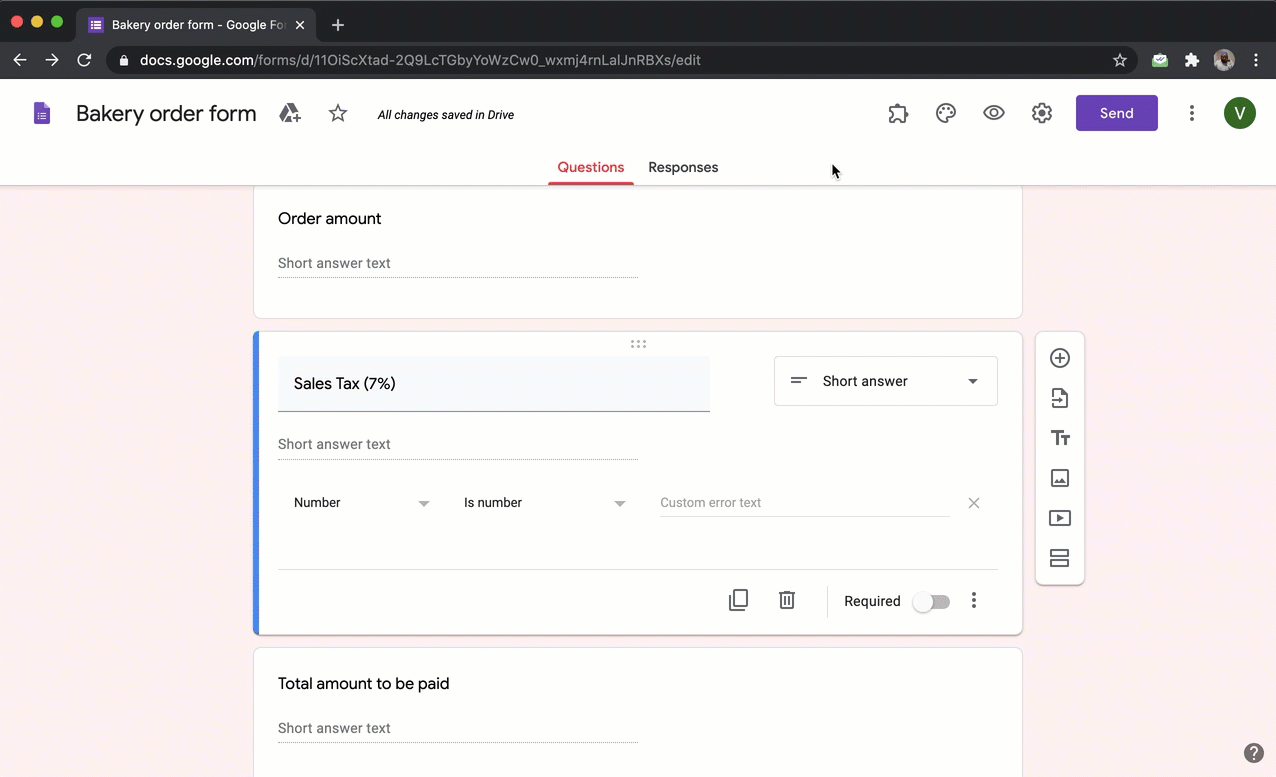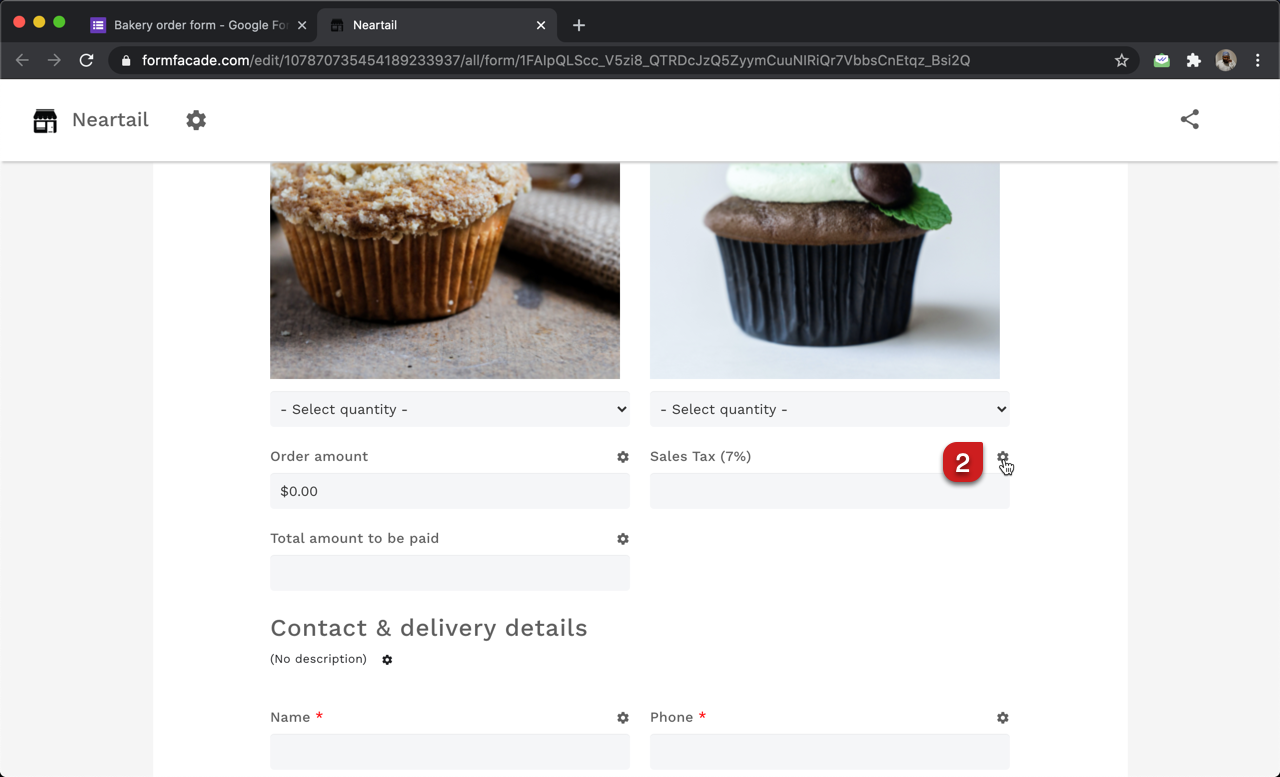#### Formula to calculate the taxes

If you know the tax rates that you need to charge your customers and pay the local tax authority, you can easily write the formula to calculate the taxes. Otherwise, you can check with your accountant to confirm the tax rates.

Let's say the sales tax is 7%. You will have to simply multiply order amount by 0.07 to calculate the tax. We can use the CURRENCY function to format and display the calculated tax as a currency.

CURRENCY("\$", Order amount * 0.07)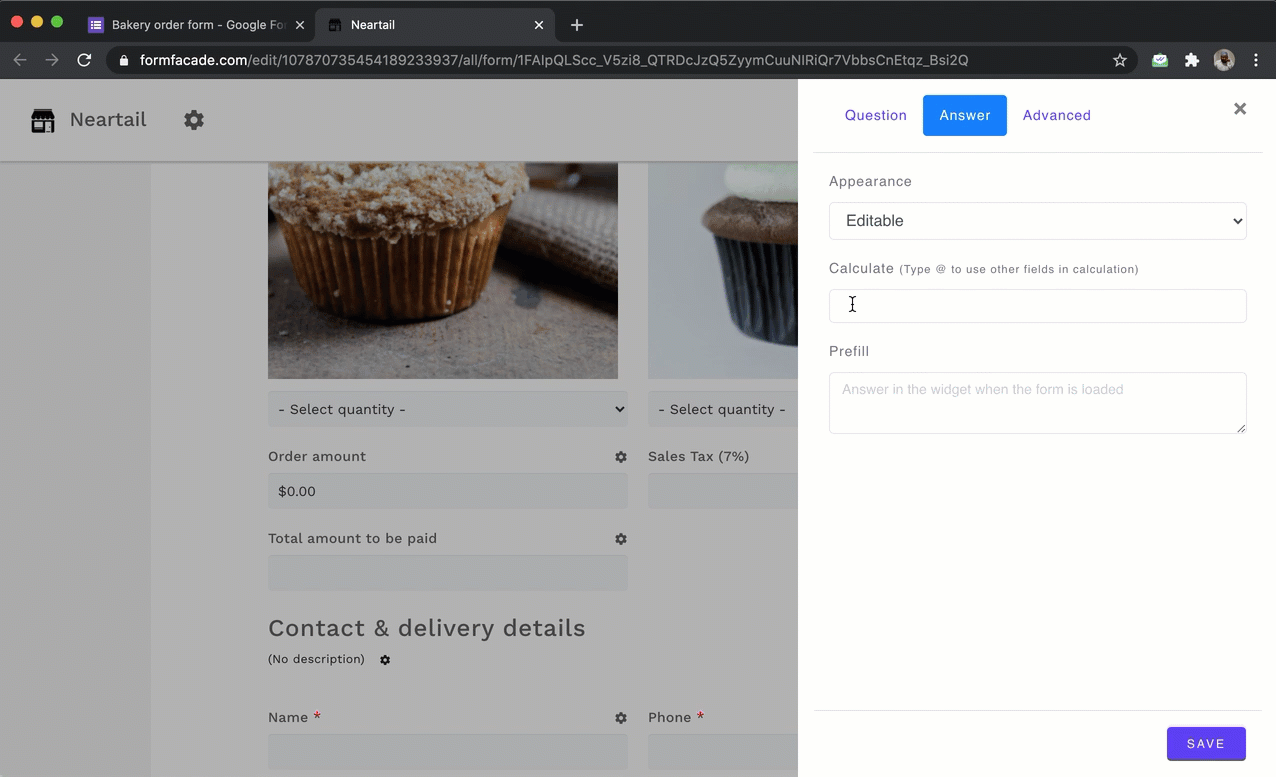#### How to calculate the total amount including taxes?